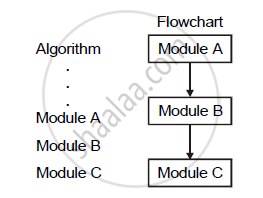HSC Science (Computer Science) 12th Board ExamMaharashtra State Board
Share

# Explain with flowchart the following control structure : Sequence Logic - HSC Science (Computer Science) 12th Board Exam - Computer Science 1

ConceptBasic Data Structures (Stack, Queue, Dequeue)

#### Question

Explain with flowchart the following control structure : Sequence Logic

#### Solution

(i) Sequence Logic:
(1) In the sequence logic modules are executed sequentially one after another.
(2) The sequence is represented by means of numbered steps. The flow pattern is as shown in figure.

3) The above three modules are executed in sequence that is one after anothIs there an error in this question or solution?

#### APPEARS IN

2016-2017 (March) (with solutions)
Question 2.1.1 | 3.00 marks
Solution Explain with flowchart the following control structure : Sequence Logic Concept: Basic Data Structures (Stack, Queue, Dequeue).
S